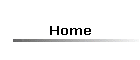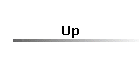# Help on Practical #1

For a chemical reaction A + B ® products, the rate is related to the concentrations of reactants by
Rate = k[A]m[B]n where m and n are normally positive integers.  Using the properties of logarithms, we have
ln(rate) = ln(k) + m ln[A] + n ln[B]
and if, for example the concentration of A, [A], is kept constant, so that ln(k) and m ln[A] are constant,
ln(rate) = const + n ln([B])
which is the equation of a straight line where ln(rate) is the dependent variable (y), ln([B]) is the independent variable (x) and n is the slope.  You must set out your results for rate and [A] in a spread sheet, calculate ln(rate) and ln([A]) and then plot ln(rate) against ln([A]), as well as rate against concentration.  Put in the line of best fit and display the relevant equation in each case.  Indicate the value of n, the order w.r.t. the reactant whose concentration was varied in your experiment.  You also need to find the rate constant.

Click on this link to view student results for the same experiment.  It shows the numerical results in full, together with the two graphs required.  In the first graph, ln(rate) is plotted against ln(concentration) and in the second one, rate is plotted against concentration, with the intercept set to zero (the rate must be zero if the concentration is zero).  Notice how the trendline for the plots is displayed.  The coefficient of x is the slope, which, in the ln/ln plot, is equal to the order of the reaction with respect to the reactant whose concentration is varied.  In the second plot, the slope is the rate constant for the reaction.  (NB. If you submit these results, you will be guilty of cheating!)

Note that all calculations are done by the spreadsheet program, you don't have to do them manually.  For example, entering the formula =ln(A2) in cell B2 finds the natural logarithm of the value in cell A2, i.e. -6.90776.  You can then copy the formula into the other cells in the column and it will be adjusted automatically to calculate the appropriate value.  Values can be entered as ordinary numbers or in scientific notation.  Excel does not recognise (nor show) 3.1×10-6, instead it expects (and may also display) 3.1E-6.

Excel draws your graphs for you.  To plot the rate against concentration, for example, select the values for the concentration with the mouse, then press Ctrl and select the values for the rate.  You should wind up with two columns of values selected.  Then click on insert and then chart followed by XY (scatter).  Then click on next and type in the chart title and the labels for the axes (you can put units in at this stage when there are any).  (Note that after taking natural logarithms (ln) the result has no units.)  You can add subscripts and superscripts later.  Click on gridlines and check all the boxes for a proper graph look.  (You can alter the spacing between the gridlines later.)  Click on legend and uncheck the box show legend.  Click next again and then finish.  When the graph shows up you can right click on each axis in turn and select format axis to adjust the range of values covered by the graph, as well as the major and minor units so as to alter the grid spacing, and various other aspects of the appearance.  Double click on a title and select a character if you want to make it a subscript, for example.  Right click on the selected character and select format chart title.  You can then select subscript (or superscript).

Now select chart and then add trend line.  You can select various types of trend line, according to theory.  Note that if you are plotting ln(rate) against ln(concentration) you should select line.  Right click on the new trend line and select format trend line then options and check the box for display equation on chart.  This latter is most useful for a straight line where you want the slope or the intercept.

You can also adjust things such as the background colour, and also the size of the chart by dragging the corners or sides.  Make sure you give the chart a title as well as each axis.

Put the names of all your group members (or just yourself if you are submitting individually) and a title at the top of your spreadsheet, too.